GFG App
Open AppBrowser
Continue

# Complete Guide On 2D Array (Matrix) Rotations – Data Structure and Algorithms Tutorial

Matrix Rotation is defined as rotating the elements of the array around its center in a clockwise or counterclockwise direction, or rotating the elements by some position in clockwise or anticlockwise or interchanging of rows and columns.

There are several ways to perform rotations on a Matrix, including using loops, using built-in functions, etc.

## Types of Matrix Rotation:

The rotation of a Matrix can be classified into the following categories:

1. Rotate the matrix 90 degrees (or multiples of 90) clockwise or counterclockwise.
2. Rotate matrix elements by 1 (or more) places to the right or left.
3. Interchange rows and columns

The details about each type are discussed below with some examples for better understanding.

## 1. Rotate the Matrix (90 degrees clockwise):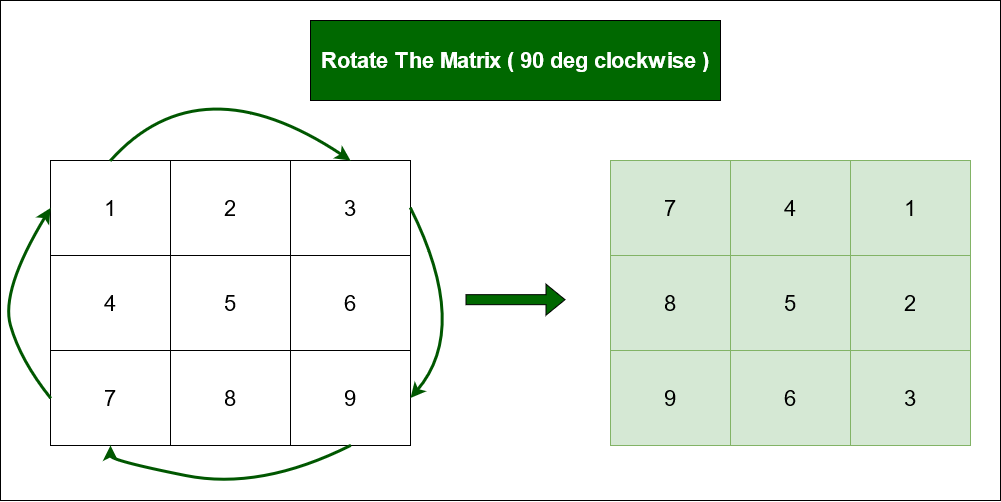Rotate the Matrix by 90 degrees clockwise

### 1: Rotate by Cycles

Intuition:

To solve the question without any extra space, rotate the array in form of squares, dividing the matrix into squares or cycles.

For example, A 4 X 4 matrix will have 2 cycles. The first cycle is formed by its 1st row, last column, last row, and 1st column. The second cycle is formed by the 2nd row, second-last column, second-last row, and 2nd column.

The idea is for each square cycle, to swap the elements involved with the corresponding cell in the matrix in an clockwise direction i.e. from top to right, right to bottom, bottom to left, and from left to top one at a time using nothing but a temporary variable to achieve this

Illustration:

Let size of row and column be 3.
During first iteration –
a[i][j] = Element at first index (leftmost corner top)= 1.
a[j][n-1-i]= Rightmost corner top Element = 3.
a[n-1-i][n-1-j] = Rightmost corner bottom element = 9.
a[n-1-j][i] = Leftmost corner bottom element = 7.
Move these elements in the clockwise direction.

During second iteration –
a[i][j] = 2.
a[j][n-1-i] = 6.
a[n-1-i][n-1-j] = 8.
a[n-1-j][i] = 4.
Similarly, move these elements in the clockwise direction.

Below is the implementation of the above approach:

## C++

 `// C++ implementation of above approach` `#include ` `using` `namespace` `std;`   `#define N 3`   `// Function to rotate the matrix 90 degree clockwise` `void` `rotate90Clockwise(``int` `a[N][N])` `{`   `    ``// Traverse each cycle` `    ``for` `(``int` `i = 0; i < N / 2; i++) {` `        ``for` `(``int` `j = i; j < N - i - 1; j++) {`   `            ``// Swap elements of each cycle` `            ``// in clockwise direction` `            ``int` `temp = a[i][j];` `            ``a[i][j] = a[N - 1 - j][i];` `            ``a[N - 1 - j][i] = a[N - 1 - i][N - 1 - j];` `            ``a[N - 1 - i][N - 1 - j] = a[j][N - 1 - i];` `            ``a[j][N - 1 - i] = temp;` `        ``}` `    ``}` `}`   `// Function for print matrix` `void` `printMatrix(``int` `arr[N][N])` `{` `    ``for` `(``int` `i = 0; i < N; i++) {` `        ``for` `(``int` `j = 0; j < N; j++)` `            ``cout << arr[i][j] << ``" "``;` `        ``cout << ``'\n'``;` `    ``}` `}`   `// Driver code` `int` `main()` `{` `    ``int` `arr[N][N]` `        ``= { { 1, 2, 3 }, { 4, 5, 6 }, { 7, 8, 9 } };` `    ``rotate90Clockwise(arr);` `    ``printMatrix(arr);` `    ``return` `0;` `}`

## Java

 `// Java implementation of above approach` `import` `java.io.*;`   `class` `GFG {`   `    ``static` `int` `N = ``3``;`   `    ``// Function to rotate the matrix 90 degree clockwise` `    ``static` `void` `rotate90Clockwise(``int` `a[][])` `    ``{`   `        ``// Traverse each cycle` `        ``for` `(``int` `i = ``0``; i < N / ``2``; i++) {` `            ``for` `(``int` `j = i; j < N - i - ``1``; j++) {`   `                ``// Swap elements of each cycle` `                ``// in clockwise direction` `                ``int` `temp = a[i][j];` `                ``a[i][j] = a[N - ``1` `- j][i];` `                ``a[N - ``1` `- j][i] = a[N - ``1` `- i][N - ``1` `- j];` `                ``a[N - ``1` `- i][N - ``1` `- j] = a[j][N - ``1` `- i];` `                ``a[j][N - ``1` `- i] = temp;` `            ``}` `        ``}` `    ``}`   `    ``// Function for print matrix` `    ``static` `void` `printMatrix(``int` `arr[][])` `    ``{` `        ``for` `(``int` `i = ``0``; i < N; i++) {` `            ``for` `(``int` `j = ``0``; j < N; j++)` `                ``System.out.print(arr[i][j] + ``" "``);` `            ``System.out.println();` `        ``}` `    ``}`   `    ``// Driver code`   `    ``public` `static` `void` `main(String[] args)` `    ``{` `        ``int` `arr[][]` `            ``= { { ``1``, ``2``, ``3` `}, { ``4``, ``5``, ``6` `}, { ``7``, ``8``, ``9` `} };` `        ``rotate90Clockwise(arr);` `        ``printMatrix(arr);` `    ``}` `}`

## Python3

 `# Python code to implement the above approach`     `# Function to rotate matrix 90 degree clockwise` `def` `rotate90Clockwise(A):` `    ``N ``=` `len``(A[``0``])` `    ``for` `i ``in` `range``(N ``/``/` `2``):` `        ``for` `j ``in` `range``(i, N ``-` `i ``-` `1``):` `            ``temp ``=` `A[i][j]` `            ``A[i][j] ``=` `A[N ``-` `1` `-` `j][i]` `            ``A[N ``-` `1` `-` `j][i] ``=` `A[N ``-` `1` `-` `i][N ``-` `1` `-` `j]` `            ``A[N ``-` `1` `-` `i][N ``-` `1` `-` `j] ``=` `A[j][N ``-` `1` `-` `i]` `            ``A[j][N ``-` `1` `-` `i] ``=` `temp`     `# Function to print the matrix` `def` `printMatrix(A):` `    ``N ``=` `len``(A[``0``])` `    ``for` `i ``in` `range``(N):` `        ``print``(A[i])`     `# Driver code` `if` `__name__ ``=``=` `'__main__'``:` `    ``A ``=` `[[``1``, ``2``, ``3``],` `         ``[``4``, ``5``, ``6``],` `         ``[``7``, ``8``, ``9``]]` `    ``rotate90Clockwise(A)` `    ``printMatrix(A)`

## C#

 `// C# implementation of above approach`   `using` `System;`   `class` `GFG {` `    ``static` `int` `N = 3;`   `    ``// Function to rotate the matrix 90 degree clockwise` `    ``static` `void` `Rotate90Clockwise(``int``[, ] a)` `    ``{`   `        ``// Traverse each cycle` `        ``for` `(``int` `i = 0; i < N / 2; i++) {` `            ``for` `(``int` `j = i; j < N - i - 1; j++) {`   `                ``// Swap elements of each cycle` `                ``// in clockwise direction` `                ``int` `temp = a[i, j];` `                ``a[i, j] = a[N - 1 - j, i];` `                ``a[N - 1 - j, i] = a[N - 1 - i, N - 1 - j];` `                ``a[N - 1 - i, N - 1 - j] = a[j, N - 1 - i];` `                ``a[j, N - 1 - i] = temp;` `            ``}` `        ``}` `    ``}`   `    ``// Function for print matrix` `    ``static` `void` `PrintMatrix(``int``[, ] arr)` `    ``{` `        ``for` `(``int` `i = 0; i < N; i++) {` `            ``for` `(``int` `j = 0; j < N; j++)` `                ``Console.Write(arr[i, j] + ``" "``);` `            ``Console.WriteLine();` `        ``}` `    ``}`   `    ``// Driver code` `    ``static` `void` `Main(``string``[] args)` `    ``{` `        ``int``[, ] arr` `            ``= { { 1, 2, 3 }, { 4, 5, 6 }, { 7, 8, 9 } };` `        ``Rotate90Clockwise(arr);` `        ``PrintMatrix(arr);` `    ``}` `}`

## Javascript

 `const N = 3;`   `// Function to rotate the matrix 90 degree clockwise` `function` `rotate90Clockwise(a) {`   `    ``// Traverse each cycle` `    ``for` `(let i = 0; i < N / 2; i++) {` `        ``for` `(let j = i; j < N - i - 1; j++) {`   `            ``// Swap elements of each cycle` `            ``// in clockwise direction` `            ``let temp = a[i][j];` `            ``a[i][j] = a[N - 1 - j][i];` `            ``a[N - 1 - j][i] = a[N - 1 - i][N - 1 - j];` `            ``a[N - 1 - i][N - 1 - j] = a[j][N - 1 - i];` `            ``a[j][N - 1 - i] = temp;` `        ``}` `    ``}` `}`   `// Function for print matrix` `function` `printMatrix(arr) {` `    ``for` `(let i = 0; i < N; i++) {` `        ``let row = ``""``;` `        ``for` `(let j = 0; j < N; j++) {` `            ``row += arr[i][j] + ``" "``;` `        ``}` `        ``console.log(row);` `    ``}` `}`   `// Driver code` `let arr = [ [ 1, 2, 3 ], [ 4, 5, 6 ], [ 7, 8, 9 ] ];` `rotate90Clockwise(arr);` `printMatrix(arr);`

Output

```7 4 1
8 5 2
9 6 3 ```

Time Complexity: O(n*n)
Auxiliary Space: O(1)

### 2: Rotate through diagonal and middle:

The Approach is to rotate the given matrix two times, first time with respect to the Main diagonal, next time rotate the resultant matrix with respect to the middle column, Consider the following illustration to have a clear insight into it.

Illustration:

Consider the following matrix.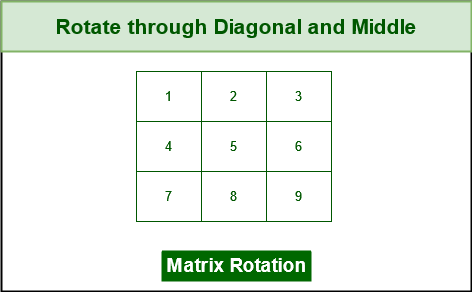Original Matrix

• Swap all elements which are on opposite sides of the main diagonal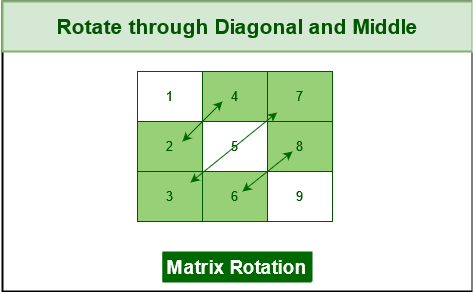Rotate through the diagonal

• Next, swap elements which are on either sides of the middle column, and we get the desired matrix.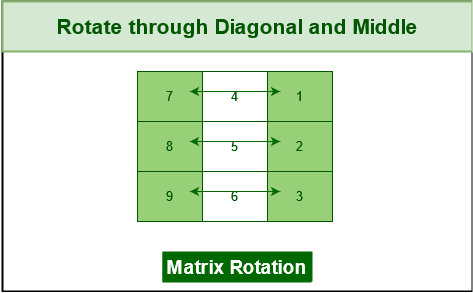Rotate through the middle

Below is the implementation of the above approach:

## C++

 `// C++ code to implement above approach` `#include ` `using` `namespace` `std;`   `#define N 3`   `void` `print(``int` `arr[N][N])` `{` `    ``for` `(``int` `i = 0; i < N; ++i) {` `        ``for` `(``int` `j = 0; j < N; ++j)` `            ``cout << arr[i][j] << ``" "``;`   `        ``cout << ``'\n'``;` `    ``}` `}`   `void` `rotate(``int` `arr[N][N])` `{`   `    ``// First rotation` `    ``// with respect to main diagonal` `    ``for` `(``int` `i = 0; i < N; ++i) {` `        ``for` `(``int` `j = 0; j < i; ++j) {` `            ``int` `temp = arr[i][j];` `            ``arr[i][j] = arr[j][i];` `            ``arr[j][i] = temp;` `        ``}` `    ``}`   `    ``// Second rotation` `    ``// with respect to middle column` `    ``for` `(``int` `i = 0; i < N; ++i) {` `        ``for` `(``int` `j = 0; j < N / 2; ++j) {` `            ``int` `temp = arr[i][j];` `            ``arr[i][j] = arr[i][N - j - 1];` `            ``arr[i][N - j - 1] = temp;` `        ``}` `    ``}` `}`   `// Driver code` `int` `main()` `{` `    ``int` `arr[N][N]` `        ``= { { 1, 2, 3 }, { 4, 5, 6 }, { 7, 8, 9 } };` `    ``rotate(arr);` `    ``print(arr);` `    ``return` `0;` `}`

## Java

 `// Java code to implement above approach` `import` `java.io.*;`   `class` `GFG {`   `    ``static` `void` `rotate(``int``[][] arr)` `    ``{`   `        ``int` `n = arr.length;`   `        ``// first rotation` `        ``// with respect to main diagonal` `        ``for` `(``int` `i = ``0``; i < n; ++i) {` `            ``for` `(``int` `j = ``0``; j < i; ++j) {` `                ``int` `temp = arr[i][j];` `                ``arr[i][j] = arr[j][i];` `                ``arr[j][i] = temp;` `            ``}` `        ``}` `        ``// Second rotation` `        ``// with respect to middle column` `        ``for` `(``int` `i = ``0``; i < n; ++i) {` `            ``for` `(``int` `j = ``0``; j < n / ``2``; ++j) {` `                ``int` `temp = arr[i][j];` `                ``arr[i][j] = arr[i][n - j - ``1``];` `                ``arr[i][n - j - ``1``] = temp;` `            ``}` `        ``}` `    ``}`   `    ``// to print matrix` `    ``static` `void` `printMatrix(``int` `arr[][])` `    ``{` `        ``int` `n = arr.length;` `        ``for` `(``int` `i = ``0``; i < n; i++) {` `            ``for` `(``int` `j = ``0``; j < n; j++)` `                ``System.out.print(arr[i][j] + ``" "``);` `            ``System.out.println();` `        ``}` `    ``}` `    ``// Driver code` `    ``public` `static` `void` `main(String[] args)` `    ``{` `        ``int` `arr[][]` `            ``= { { ``1``, ``2``, ``3` `}, { ``4``, ``5``, ``6` `}, { ``7``, ``8``, ``9` `} };` `        ``rotate(arr);` `        ``printMatrix(arr);` `    ``}` `}`

## Python3

 `# Python3 implementation of above approach` `N ``=` `3`     `# Function to rotate the matrix 90 degree clockwise` `def` `rotate(arr):` `    ``global` `N`   `    ``# First rotation` `    ``# with respect to main diagonal` `    ``for` `i ``in` `range``(N):` `        ``for` `j ``in` `range``(i):` `            ``temp ``=` `arr[i][j]` `            ``arr[i][j] ``=` `arr[j][i]` `            ``arr[j][i] ``=` `temp`   `    ``# Second rotation` `    ``# with respect to middle column` `    ``for` `i ``in` `range``(N):` `        ``for` `j ``in` `range``(``int``(N``/``2``)):` `            ``temp ``=` `arr[i][j]` `            ``arr[i][j] ``=` `arr[i][N``-``j``-``1``]` `            ``arr[i][N``-``j``-``1``] ``=` `temp`     `# Driver code` `if` `__name__ ``=``=` `'__main__'``:` `    ``arr ``=` `[[``1``, ``2``, ``3``],` `           ``[``4``, ``5``, ``6``],` `           ``[``7``, ``8``, ``9``]]` `    `  `    ``rotate(arr)` `    `  `    ``for` `i ``in` `range``(N):` `        ``for` `j ``in` `range``(N):` `            ``print``(arr[i][j], end``=``" "``)` `        ``print``()`

## C#

 `// C# code to implement above approach`   `using` `System;`   `class` `GFG {` `    ``static` `int` `N = 3;` `  `  `    ``// Driver's code` `    ``static` `void` `Main()` `    ``{` `          ``// Input array` `        ``int``[,] arr = { { 1, 2, 3 }, { 4, 5, 6 }, { 7, 8, 9 } };`   `          ``// Function call` `        ``Rotate(arr);` `        ``Print(arr);` `    ``}`   `      ``// Function to rotate the matrix 90 degree clockwise` `    ``static` `void` `Rotate(``int``[,] arr)` `    ``{` `        ``// First rotation with respect to main diagonal` `        ``for` `(``int` `i = 0; i < N; ++i)` `        ``{` `            ``for` `(``int` `j = 0; j < i; ++j)` `            ``{` `                ``int` `temp = arr[i,j];` `                ``arr[i,j] = arr[j,i];` `                ``arr[j,i] = temp;` `            ``}` `        ``}`   `        ``// Second rotation with respect to middle column` `        ``for` `(``int` `i = 0; i < N; ++i)` `        ``{` `            ``for` `(``int` `j = 0; j < N / 2; ++j)` `            ``{` `                ``int` `temp = arr[i,j];` `                ``arr[i,j] = arr[i,N - j - 1];` `                ``arr[i,N - j - 1] = temp;` `            ``}` `        ``}` `    ``}` `    `  `      ``// Function to print the final array` `    ``static` `void` `Print(``int``[,] arr)` `    ``{` `        ``for` `(``int` `i = 0; i < N; ++i)` `        ``{` `            ``for` `(``int` `j = 0; j < N; ++j)` `                ``Console.Write(arr[i,j] + ``" "``);`   `            ``Console.WriteLine();` `        ``}` `    ``}` `}`

## Javascript

 `// JavaScript code to implement above approach`   `const N = 3;`   `// function to print the matrix` `function` `print(arr)` `{` `    ``for` `(let i = 0; i < N; i++) {` `        ``let row = ``""``;` `        ``for` `(let j = 0; j < N; j++) {` `            ``row += arr[i][j] + ``" "``;` `        ``}` `        ``console.log(row);` `    ``}` `}`   `// function to rotate the matrix` `function` `rotate(arr)` `{` `    ``// First rotation with respect to main diagonal` `    ``for` `(let i = 0; i < N; ++i) {` `        ``for` `(let j = 0; j < i; ++j) {` `            ``let temp = arr[i][j];` `            ``arr[i][j] = arr[j][i];` `            ``arr[j][i] = temp;` `        ``}` `    ``}`   `    ``// Second rotation with respect to middle column` `    ``for` `(let i = 0; i < N; ++i) {` `        ``for` `(let j = 0; j < N / 2; ++j) {` `            ``let temp = arr[i][j];` `            ``arr[i][j] = arr[i][N - j - 1];` `            ``arr[i][N - j - 1] = temp;` `        ``}` `    ``}` `}`   `// Driver code` `let arr = [ [ 1, 2, 3 ], [ 4, 5, 6 ], [ 7, 8, 9 ] ];` `rotate(arr);` `print(arr);`

Output

```7 4 1
8 5 2
9 6 3 ```

Time Complexity: O(n * n)
Auxiliary Space: O(1)

## 2. Rotate Matrix elements by one place:

• We have the original matrix.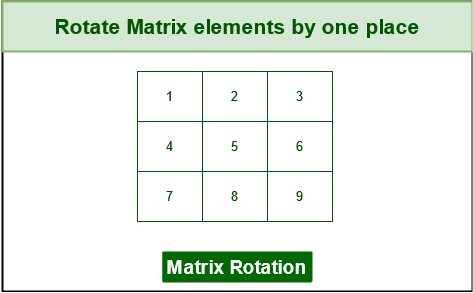Rotate matrix elements by one place

• Rotate by one place to the right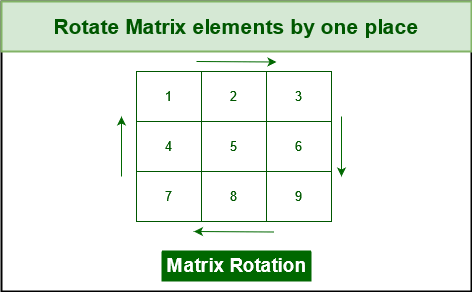Rotate matrix elements by one place

• Final matrix after the shifts.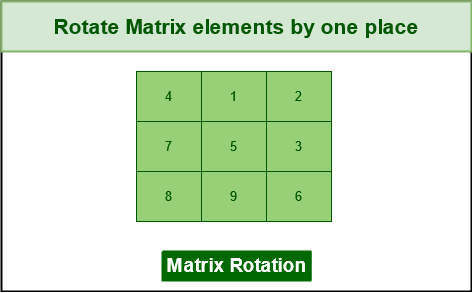Rotate matrix elements by one place

Intuition:

The idea is to use loops similar to the program for printing a matrix in spiral form
One by one rotate all rings of elements, starting from the outermost. To rotate a ring, we need to do the following.

• Move elements of top row.
• Move elements of last column.
• Move elements of bottom row.
• Move elements of first column.
• Repeat above steps for inner ring while there is an inner ring.

Below is the implementation of the above approach:

## C++

 `// C++ program to implement the above approach`   `#include ` `#define R 3` `#define C 3` `using` `namespace` `std;`   `// A function to rotate a matrix mat[][] of size R x C.` `// Initially, m = R and n = C` `void` `rotateMatrix(``int` `m, ``int` `n, ``int` `mat[R][C])` `{` `    ``int` `row = 0, col = 0;` `    ``int` `prev, curr;`   `    ``while` `(row < m && col < n) {`   `        ``if` `(row + 1 == m || col + 1 == n)` `            ``break``;`   `        ``// Store the first element of next row, this` `        ``// element will replace first element of current` `        ``// row` `        ``prev = mat[row + 1][col];`   `        ``// Move elements of first row ` `        ``// from the remaining rows ` `        ``for` `(``int` `i = col; i < n; i++) {` `            ``curr = mat[row][i];` `            ``mat[row][i] = prev;` `            ``prev = curr;` `        ``}` `        ``row++;`   `        ``// Move elements of last column ` `        ``// from the remaining columns` `        ``for` `(``int` `i = row; i < m; i++) {` `            ``curr = mat[i][n - 1];` `            ``mat[i][n - 1] = prev;` `            ``prev = curr;` `        ``}` `        ``n--;`   `        ``// Move elements of last row ` `        ``// from the remaining rows` `        ``if` `(row < m) {` `            ``for` `(``int` `i = n - 1; i >= col; i--) {` `                ``curr = mat[m - 1][i];` `                ``mat[m - 1][i] = prev;` `                ``prev = curr;` `            ``}` `        ``}` `        ``m--;`   `        ``// Move elements of first column ` `        ``// from the remaining rows` `        ``if` `(col < n) {` `            ``for` `(``int` `i = m - 1; i >= row; i--) {` `                ``curr = mat[i][col];` `                ``mat[i][col] = prev;` `                ``prev = curr;` `            ``}` `        ``}` `        ``col++;` `    ``}`   `    ``// Print rotated matrix` `    ``for` `(``int` `i = 0; i < R; i++) {` `        ``for` `(``int` `j = 0; j < C; j++)` `            ``cout << mat[i][j] << ``" "``;` `        ``cout << endl;` `    ``}` `}`   `// Driver code` `int` `main()` `{` `    ``// Input Matrix` `    ``int` `a[R][C] = { { 1, 2, 3 }, { 4, 5, 6 }, { 7, 8, 9 } };`   `    ``rotateMatrix(R, C, a);` `    ``return` `0;` `}`

## Java

 `// Java program to implement above approach` `import` `java.lang.*;` `import` `java.util.*;`   `class` `GFG {` `    ``static` `int` `R = ``3``;` `    ``static` `int` `C = ``3``;`   `    ``// A function to rotate a matrix` `    ``// mat[][] of size R x C.` `    ``// Initially, m = R and n = C` `    ``static` `void` `rotateMatrix(``int` `m, ``int` `n, ``int` `mat[][])` `    ``{` `        ``int` `row = ``0``, col = ``0``;` `        ``int` `prev, curr;`   `        ``/*` `        ``row - Starting row index` `        ``m - ending row index` `        ``col - starting column index` `        ``n - ending column index` `        ``i - iterator` `        ``*/` `        ``while` `(row < m && col < n) {`   `            ``if` `(row + ``1` `== m || col + ``1` `== n)` `                ``break``;`   `            ``// Store the first element of next` `            ``// row, this element will replace` `            ``// first element of current row` `            ``prev = mat[row + ``1``][col];`   `            ``// Move elements of first row` `            ``// from the remaining rows` `            ``for` `(``int` `i = col; i < n; i++) {` `                ``curr = mat[row][i];` `                ``mat[row][i] = prev;` `                ``prev = curr;` `            ``}` `            ``row++;`   `            ``// Move elements of last column` `            ``// from the remaining columns` `            ``for` `(``int` `i = row; i < m; i++) {` `                ``curr = mat[i][n - ``1``];` `                ``mat[i][n - ``1``] = prev;` `                ``prev = curr;` `            ``}` `            ``n--;`   `            ``// Move elements of last row` `            ``// from the remaining rows` `            ``if` `(row < m) {` `                ``for` `(``int` `i = n - ``1``; i >= col; i--) {` `                    ``curr = mat[m - ``1``][i];` `                    ``mat[m - ``1``][i] = prev;` `                    ``prev = curr;` `                ``}` `            ``}` `            ``m--;`   `            ``// Move elements of first column` `            ``// from the remaining rows` `            ``if` `(col < n) {` `                ``for` `(``int` `i = m - ``1``; i >= row; i--) {` `                    ``curr = mat[i][col];` `                    ``mat[i][col] = prev;` `                    ``prev = curr;` `                ``}` `            ``}` `            ``col++;` `        ``}`   `        ``// Print rotated matrix` `        ``for` `(``int` `i = ``0``; i < R; i++) {` `            ``for` `(``int` `j = ``0``; j < C; j++)` `                ``System.out.print(mat[i][j] + ``" "``);` `            ``System.out.print(``"\n"``);` `        ``}` `    ``}`   `    ``/* Driver program to test above functions */` `    ``public` `static` `void` `main(String[] args)` `    ``{` `        ``// input matrix` `        ``int` `a[][]` `            ``= { { ``1``, ``2``, ``3` `}, { ``4``, ``5``, ``6` `}, { ``7``, ``8``, ``9` `} };`   `        ``rotateMatrix(R, C, a);` `    ``}` `}`

## Python3

 `# Python program to implement above approach`     `# Function to rotate a matrix` `def` `rotateMatrix(mat):`   `    ``if` `not` `len``(mat):` `        ``return` `    ``top ``=` `0` `    ``bottom ``=` `len``(mat)``-``1`   `    ``left ``=` `0` `    ``right ``=` `len``(mat[``0``])``-``1`   `    ``while` `left < right ``and` `top < bottom:`   `        ``# Store the first element of next row,` `        ``# this element will replace first element of` `        ``# current row` `        ``prev ``=` `mat[top``+``1``][left]`   `        ``# Move elements of top row one step right` `        ``for` `i ``in` `range``(left, right``+``1``):` `            ``curr ``=` `mat[top][i]` `            ``mat[top][i] ``=` `prev` `            ``prev ``=` `curr`   `        ``top ``+``=` `1`   `        ``# Move elements of rightmost column one step downwards` `        ``for` `i ``in` `range``(top, bottom``+``1``):` `            ``curr ``=` `mat[i][right]` `            ``mat[i][right] ``=` `prev` `            ``prev ``=` `curr`   `        ``right ``-``=` `1`   `        ``# Move elements of bottom row one step left` `        ``for` `i ``in` `range``(right, left``-``1``, ``-``1``):` `            ``curr ``=` `mat[bottom][i]` `            ``mat[bottom][i] ``=` `prev` `            ``prev ``=` `curr`   `        ``bottom ``-``=` `1`   `        ``# Move elements of leftmost column one step upwards` `        ``for` `i ``in` `range``(bottom, top``-``1``, ``-``1``):` `            ``curr ``=` `mat[i][left]` `            ``mat[i][left] ``=` `prev` `            ``prev ``=` `curr`   `        ``left ``+``=` `1`   `    ``return` `mat`     `# Function to print matrix` `def` `printMatrix(mat):` `    ``for` `row ``in` `mat:` `        ``print``(row)`     `# Driver code` `if` `__name__ ``=``=` `'__main__'``:` `    ``matrix ``=` `[[``1``, ``2``, ``3``], ` `              ``[``4``, ``5``, ``6``], ` `              ``[``7``, ``8``, ``9``]]` `    ``matrix ``=` `rotateMatrix(matrix)` `    `  `    ``# Print modified matrix` `    ``printMatrix(matrix)`

## C#

 `// C# program to implement the above approach`   `using` `System;`   `class` `GFG {` `    ``static` `int` `R = 3;` `    ``static` `int` `C = 3;` `  `  `    ``// A function to rotate a matrix mat[][] of size R x C.` `    ``// Initially, m = R and n = C` `    ``static` `void` `RotateMatrix(``int``[,] mat, ``int` `m, ``int` `n) {` `        ``int` `row = 0, col = 0;` `        ``int` `prev, curr;`   `        ``while` `(row < m && col < n) {` `            ``if` `(row + 1 == m || col + 1 == n)` `                ``break``;`   `            ``// Store the first element of next row, this` `            ``// element will replace first element of current` `            ``// row` `            ``prev = mat[row + 1, col];`   `            ``// Move elements of first row` `            ``// from the remaining rows` `            ``for` `(``int` `i = col; i < n; i++) {` `                ``curr = mat[row, i];` `                ``mat[row, i] = prev;` `                ``prev = curr;` `            ``}` `          `  `            ``row++;` `    `  `            ``// Move elements of last column` `            ``// from the remaining columns` `            ``for` `(``int` `i = row; i < m; i++) {` `                ``curr = mat[i, n - 1];` `                ``mat[i, n - 1] = prev;` `                ``prev = curr;` `            ``}` `          `  `            ``n--;` `        `  `            ``// Move elements of last row` `            ``// from the remaining rows` `            ``if` `(row < m) {` `                ``for` `(``int` `i = n - 1; i >= col; i--) {` `                    ``curr = mat[m - 1, i];` `                    ``mat[m - 1, i] = prev;` `                    ``prev = curr;` `                ``}` `            ``}` `          `  `            ``m--;` `    `  `              ``// Move elements of first column` `            ``// from the remaining rows` `            ``if` `(col < n) {` `                ``for` `(``int` `i = m - 1; i >= row; i--) {` `                    ``curr = mat[i, col];` `                    ``mat[i, col] = prev;` `                    ``prev = curr;` `                ``}` `            ``}` `          `  `            ``col++;` `        ``}`   `        ``// Print rotated matrix` `        ``for` `(``int` `i = 0; i < R; i++) {` `            ``for` `(``int` `j = 0; j < C; j++) {` `                ``Console.Write(mat[i, j] + ``" "``);` `            ``}` `          `  `            ``Console.WriteLine();` `        ``}` `    ``}` `    `  `      ``// Driver's code` `    ``static` `void` `Main(``string``[] args) {` `        ``// Input Matrix` `        ``int``[,] mat = { { 1, 2, 3 }, { 4, 5, 6 }, { 7, 8, 9 } };`   `        ``RotateMatrix(mat, R, C);` `    ``}` `}`

Output

```4 1 2
7 5 3
8 9 6 ```

Time Complexity: O(m*n) where m is the number of rows & n is the number of columns.
Auxiliary Space: O(1).

## 3. Rotations in Matrix by row or column ( Transpose of the matrix ):

Transpose of a matrix is obtained by changing rows to columns and columns to rows. In other words, the transpose of A[N][M] is obtained by changing A[i][j] to A[j][i].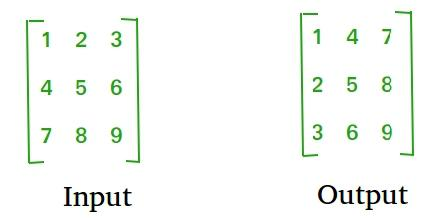Transpose of the matrix

There are two methods to solve this kind of problem:

### 1. Using Extra Space

Intuition:

Run a nested loop using two integer pointers i and j for 0 <= i < N and 0 <= j < M
Set B[i][j] equal to A[j][i]

Below is the implementation of the above approach:

## C++

 `// C++ program to implement the above approach` `#include ` `using` `namespace` `std;` `#define N 3`   `// This function stores transpose` `// of A[][] in B[][]` `void` `transpose(``int` `A[][N], ``int` `B[][N])` `{` `    ``int` `i, j;` `    ``for` `(i = 0; i < N; i++)` `        ``for` `(j = 0; j < N; j++)` `            ``B[i][j] = A[j][i];` `}`   `// Driver code` `int` `main()` `{` `    ``int` `A[N][N] = { { 1, 2, 3 }, { 4, 5, 6 }, { 7, 8, 9 } };`   `    ``// Note dimensions of B[][]` `    ``int` `B[N][N], i, j;`   `    ``// Function call` `    ``transpose(A, B);`   `    ``cout << ``"Modified matrix is \n"``;` `    ``for` `(i = 0; i < N; i++) {` `        ``for` `(j = 0; j < N; j++)` `            ``cout << ``" "` `<< B[i][j];`   `        ``cout << ``"\n"``;` `    ``}` `    ``return` `0;` `}`

## Java

 `// Java Program to implement the above approach`   `class` `GFG {` `    ``static` `final` `int` `N = ``3``;`   `    ``// This function stores transpose` `    ``// of A[][] in B[][]` `    ``static` `void` `transpose(``int` `A[][], ``int` `B[][])` `    ``{` `        ``int` `i, j;` `        ``for` `(i = ``0``; i < N; i++)` `            ``for` `(j = ``0``; j < N; j++)` `                ``B[i][j] = A[j][i];` `    ``}`   `    ``// Driver code` `    ``public` `static` `void` `main(String[] args)` `    ``{` `        ``int` `A[][]` `            ``= { { ``1``, ``2``, ``3` `}, { ``4``, ``5``, ``6` `}, { ``7``, ``8``, ``9` `} };`   `        ``int` `B[][] = ``new` `int``[N][N], i, j;`   `        ``// Function call` `        ``transpose(A, B);`   `        ``System.out.print(``"Modified matrix is \n"``);` `        ``for` `(i = ``0``; i < N; i++) {` `            ``for` `(j = ``0``; j < N; j++)` `                ``System.out.print(B[i][j] + ``" "``);` `            ``System.out.print(``"\n"``);` `        ``}` `    ``}` `}`

## Python3

 `# Python3 code to implement the above approach`   `N ``=` `3`     `# This function stores` `# transpose of A[][] in B[][]` `def` `transpose(A, B):` `    ``for` `i ``in` `range``(N):` `        ``for` `j ``in` `range``(N):` `            ``B[i][j] ``=` `A[j][i]`     `# Driver code` `if` `__name__ ``=``=` `'__main__'``:` `    ``A ``=` `[[``1``, ``2``, ``3``],` `         ``[``4``, ``5``, ``6``],` `         ``[``7``, ``8``, ``9``]]` `    `  `    ``# To store result` `    ``B ``=` `[[``0` `for` `x ``in` `range``(N)] ``for` `y ``in` `range``(N)]` `    `  `    ``# Function call` `    ``transpose(A, B)` `    `  `    ``print``(``"Modified matrix is"``)` `    ``for` `i ``in` `range``(N):` `        ``for` `j ``in` `range``(N):` `            ``print``(B[i][j], ``" "``, end``=``'')` `        ``print``()`

Output

```Modified matrix is
1 4 7
2 5 8
3 6 9```

Time complexity: O(M x N).
Auxiliary Space: O(M x N), since M x N extra space has been used.

### 2. Using Constant Space:

Run a nested loop using two integer pointers i and j for 0 <= i < N and 0 <= j < M.
Swap A[i][j] with A[j][i].

Below is the implementation of the above approach:

## C++

 `// C++ code to implement the above approach` `#include ` `using` `namespace` `std;`   `#define N 3`   `// Converts A[][] to its transpose` `void` `transpose(``int` `A[][N])` `{` `    ``for` `(``int` `i = 0; i < N; i++)` `        ``for` `(``int` `j = i + 1; j < N; j++)` `            ``swap(A[i][j], A[j][i]);` `}`   `int` `main()` `{` `    ``int` `A[N][N] = { { 1, 2, 3 }, { 4, 5, 6 }, { 7, 8, 9 } };`   `    ``transpose(A);`   `    ``printf``(``"Modified matrix is \n"``);` `    ``for` `(``int` `i = 0; i < N; i++) {` `        ``for` `(``int` `j = 0; j < N; j++)` `            ``printf``(``"%d "``, A[i][j]);` `        ``printf``(``"\n"``);` `    ``}`   `    ``return` `0;` `}`

## Java

 `// Java Program to implement the above approach`   `class` `GFG {` `    ``static` `final` `int` `N = ``3``;`   `    ``// Finds transpose of A[][] in-place` `    ``static` `void` `transpose(``int` `A[][])` `    ``{` `        ``for` `(``int` `i = ``0``; i < N; i++)` `            ``for` `(``int` `j = i + ``1``; j < N; j++) {` `                ``int` `temp = A[i][j];` `                ``A[i][j] = A[j][i];` `                ``A[j][i] = temp;` `            ``}` `    ``}`   `    ``// Driver code` `    ``public` `static` `void` `main(String[] args)` `    ``{` `        ``int` `A[][]` `            ``= { { ``1``, ``2``, ``3` `}, { ``4``, ``5``, ``6` `}, { ``7``, ``8``, ``9` `} };`   `        ``transpose(A);`   `        ``System.out.print(``"Modified matrix is \n"``);` `        ``for` `(``int` `i = ``0``; i < N; i++) {` `            ``for` `(``int` `j = ``0``; j < N; j++)` `                ``System.out.print(A[i][j] + ``" "``);` `            ``System.out.print(``"\n"``);` `        ``}` `    ``}` `}`

## Python3

 `# Python3 Program to implement the above approach`   `N ``=` `3`     `# Finds transpose of A[][] in-place` `def` `transpose(A):` `    ``for` `i ``in` `range``(N):` `        ``for` `j ``in` `range``(i``+``1``, N):` `            ``A[i][j], A[j][i] ``=` `A[j][i], A[i][j]`     `# Driver code` `if` `__name__ ``=``=` `'__main__'``:` `    ``A ``=` `[[``1``, ``2``, ``3``],` `         ``[``4``, ``5``, ``6``],` `         ``[``7``, ``8``, ``9``]]` `    `  `    ``transpose(A)` `    ``print``(``"Modified matrix is"``)` `    ``for` `i ``in` `range``(N):` `        ``for` `j ``in` `range``(N):` `            ``print``(A[i][j],end``=``' '``)` `        ``print``()`

Output

```Modified matrix is
1 4 7
2 5 8
3 6 9 ```

Time complexity: O(N2)
Auxiliary Space: O(1)

## Applications of Matrix Rotation:

They are useful in a variety of applications, broadly speaking, we have:-

• Image processing: In image processing, matrix rotation is often useful for rotating images to a different orientation. For example, for making an image from landscape to portrait or vice-versa.
• Data analysis: Rotating a 2D array can be useful in data analysis when visualizing or analyzing data. For example, rotating a heatmap or scatterplot can help to better understand patterns or relationships in the data.
• Computer graphics: Rotating a 2D array is useful in computer graphics for transforming objects and scenes in a 2D space. For example, rotating a 2D array of vertices can help to create animations or 2D games.

## Some FAQs regarding Matrix Rotation:

### 1. What is the time complexity of rotating a matrix?

The time complexity of rotating a 2D matrix by 90 degrees is O(N^2), N being the number of elements in the array.

### 2. Can you rotate a matrix in-place?

To rotate a matrix in-place, you can perform a series of swaps on the elements in the matrix. For example, to rotate a matrix by 90 degrees, you can swap the elements in the first row with the elements in the last column, the elements in the second row with the elements in the second-to-last column, and so on. Then, you can reverse the rows of the matrix to get the final rotated matrix.

### 3. Can I rotate the matrix by some arbitrary angle other than multiples of 90?

No, you cannot rotate a matrix by an arbitrary angle using simple operations like swapping or reversing. To rotate a matrix by an arbitrary angle, you would need to use some more complex mathematical operations like rotation matrices or complex numbers.

### 4. What is the difference between clockwise and counterclockwise rotation?

Clockwise rotation means rotating the matrix to the right, while counterclockwise rotation means rotating the matrix to the left. Because of this, they are called as right and left rotation respectively.

## Advantages of Rotations in a Matrix:

Rotating an array can have several advantages depending on the context in which it is used. Here are some potential benefits of rotating an array:

• Optimization: Rotating a 2D matrix can help to optimize certain algorithms or operations. For example, rotating a matrix can help to improve the performance of image processing algorithms, as it allows for better utilization of the cache memory.
• Better visualization: Rotating a 2D array can make it easier to visualize or analyze the data it contains. This can be particularly useful in data analysis, image processing, or other applications where visualization is important.
• Data encryption: Rotating a matrix can be used as a method of encryption in cryptography, where the elements of the array are replaced with their rotated positions based on a certain algorithm or key. This can help to make the data more secure.

## Conclusion:

In conclusion, 2D array rotation is a commonly used technique in various applications, including data visualization, data analysis, image processing, and computer graphics. When we rotate a 2D array, we can gain new insights into the data or optimizing certain algorithms or operations. However, the time complexity of rotating a 2D array can be O(N^2), where N is the number of elements in the array, which can be computationally expensive for large arrays. Overall, understanding 2D array rotation and its applications can be beneficial for various data-related tasks and problem-solving.

My Personal Notes arrow_drop_up